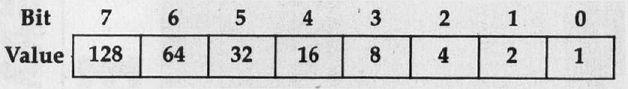COMPUTE! ISSUE 51 / AUGUST 1984 / PAGE 10

 Confusing POKEs When typing in your programs, I have sometimes come upon statements involving POKEing in the PEEK of the same POKE. I would like to know how this works. I also don't understand POKEing the PEEK while ANDing/ORing another number such as: 10 POKE 12345, PEEK(12345) OR 67 To add to my confusion would be an IF PEEK statement THEN command, that is: 20 IF PEEK (12345) THEN GOTO 67 or: 30 IF 9 AND PEEK(12345) THEN GOTO 67 Can you explain this for me? Dwight Weese Usually, when you see a POKE and a PEEK to and from the same byte, it's done in conjunction with an AND or OR command. Each byte is composed of eight bits. Each of the eight bits is like a light switch: It can be either on (1) or off (0). Each of the eight bits has its own value (see illustration below).Any byte can hold a value from 0 to 255. The value of the byte is determined by which bits are on or off, and is derived by adding the values of each of the on bits. This is called a binary number. For example, a byte containing a value of 1 would look like: 00000001 (only the 1 bit is on) A decimal eleven would look like 00001011 (the 8 plus 2 plus 1 bits are on, so: 8+2 + 1 = 11), and a byte whose value is 255 would be 11111111 (128+64 + 32 + 16+8+4+2 + 1 = 255). When you use the AND and OR commands, it's like placing a mask over the eight bits in the byte. The mask is, in effect, placed over the byte, and the bits in the byte are turned on or off according to the rules governing AND and OR. The mask can be thought of as an imaginary byte with bits set or not depending 6n the mask number. The bit pattern of the masking byte follows the same rules of binary numbers described above. For example, if you're ANDing or ORing with a value of 21, the bit pattern of the mask would look like 00010101 (16 + 4 + 1 = 21). When you AND a byte, you compare each bit of the byte with each bit of the mask. The result will be an "on bit" only if both bits (of the byte and the mask) are "on" in that position. For example, ANDing a byte with a value of eleven (00001011) with a three (00000011) would result in 00000011 (three). This is because the 1 and 2 bits of both bytes were l's, but the 8 bit in the mask was a 0. ORing a byte compares each bit in the same manner as the AND. But when you OR, if the bit in either the original or the mask byte is a one, then the result is a one ("on"). For example, ORing a byte containing a value of 15 (00001111) with 240 (11110000) would result in 11111111 (255). This is because in all cases of the compare, at least one of the bits was a 1. In your other examples, IF PEEK (12345) THEN GOTO 67 is a standard IF-THEN compare. However, in this case there is no comparing expression as in: IF PEEK (12345) =1 THEN GOTO 67. When compares are done in this manner, it is a special kind of test. In this example, the branch to 67 would be taken if the PEEKed number is anything other than zero. If the number is a zero, the test fails and there will be no GOTO. The third example, IF 9 AND PEEK (12345) THEN GOTO 67, is the same. In such cases, all numbers will answer "yes" to the IF, except zero, which will answer "no." The IF 9 AND PEEK(12345) expression will be zero in those cases when the 1 and 8 bits of location 12345 are both zero.